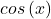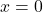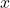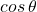# C Program To Compute Cosine Series

The program to compute cosine series is based on the mathematical concept of sequences and series – particularly power series.

The program is compiled using Dev-C++ compiler, but you can use any standard C compiler to compile and run the program. Make sure that you change the source code appropriately according to your compiler specifications.

To understand this example, you must be familiar with following C programming concepts.

## Problem Definition

Cosine series is a power series known as the Maclaurin expansion ofwhere x is a real number value. Given the value of x, the series will converge and give a finite value (radius of convergence) for the series.

We won’t be discussing, how terms of the series are generated because that would be topic for another post. The Maclaurin expansion foris for, however, it is true for all real values of.

In other words, it is same as finding the value of trigonometric ratio of, the cosine series will give you a better approximate value of.

## Program Code

/* Program to compute cosine series */
/* cos(x) = 1-x^2/2!+x^4/4!+x^6/6!+...+x^n/n! */
#include <stdio.h>
#include <stdlib.h>
#include <math.h>
main()
{
float x, t, sum;
int d;
int i, n=20;
/* Read the Input x value in degrees */
printf ("Input X Value (in degrees) :");
scanf ("%f", &x);
d= x;
x=x*3.1412/180;
t=1;
sum=1;
for (i=1; i<n+1; i++)
{
t=t*pow ((double) (-1), (double) (2*i-1))*x*x/ (2*i*(2*i-1));
sum=sum+t;
}
/* Print the Results */
for (i =0; i<35; i++)
printf ("_"); printf ("\n\n");
printf ("COS (%d) =%7.3f\n\n", (int) d, sum);
for (i =0; i<35; i++)
printf ("_"); printf ("\n\n");
system ("PAUSE");
return 0;
}

## Output

           Input X Values(in degrees):34
-------------------------------------
COS(34)= 0.829
-------------------------------------